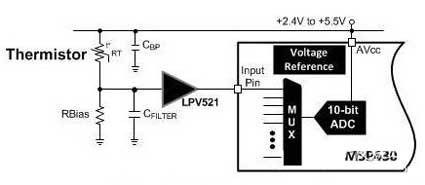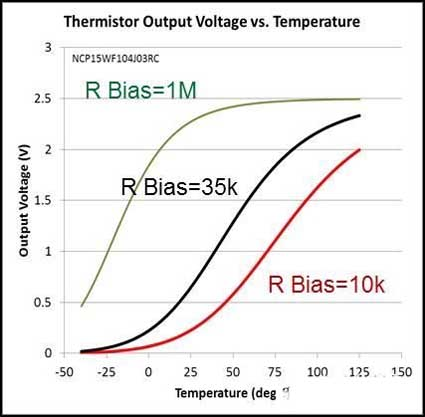﻿ Thermistor temperature sensing circuit design solution_

China sensor manufacturers

# Thermistor temperature sensing circuit design solution

Choosing the right temperature sensor not only saves money, but also maximizes system performance. In this blog post, I will mainly talk about thermistors and analog temperature sensors, both of which are cost effective temperature sensing solutions. The question is, how do you know which one to choose?
Technically, a thermistor is a resistor whose resistance changes with temperature. As shown in Figure 1, a bias circuit and a few external components are required, where the bias resistor and the thermistor form a voltage divider and are connected to an optional operational amplifier. The amplifier is connected to an analog-to-digital converter (ADC) of a microcontroller (MCU) to convert the resistance value of the thermistor into a temperature value.Figure 1 The structure diagram of the thermistor bias circuit and external components designed by Yaxun Electronics

The advantage of the thermistor is its low cost. In addition, as a resistor, it can be housed in a very small two-terminal package and placed in a wired probe.
The disadvantage of the thermistor is that its output is linear and accurate only over a narrow temperature range, and becomes non-linear outside of this range. Figure 2 plots the output curves of three thermistors with biasing resistances of 1 MΩ, 35 kΩ, and 10 kΩ, respectively. Each curve is linear over a narrow temperature range, but the three curves are non-linear when the bias resistance is 1 MΩ, 35 kΩ, and 10 kΩ, respectively, and the temperatures are 10oC, 75oC, and 50oC, respectively. Although a specially calibrated thermistor can be used to achieve the target accuracy for a specific temperature, the thermistor is costly, and the three different devices also make inventory management too complicated. Other disadvantages include unpredictable failures, low immunity, and high power consumption.figure 2: The relationship between the thermistor output voltage and temperature

For some reason, where unpredictability and power consumption are not critical, thermistors are best suited for temperature measurements over a narrow temperature range. An analog temperature sensor is an active semiconductor device whose output is a voltage or current value proportional to temperature. As an integrated circuit (IC), the analog temperature sensor has built-in intelligence that is not available in the thermistor, making design work related to analog temperature sensors simple.

﻿
﻿